Chapter 5 Temperature, Kinetic Theory, and the Gas Laws

# 5.10 Conduction

### Summary

• Calculate thermal conductivity.
• Observe conduction of heat in collisions.
• Study thermal conductivities of common substances.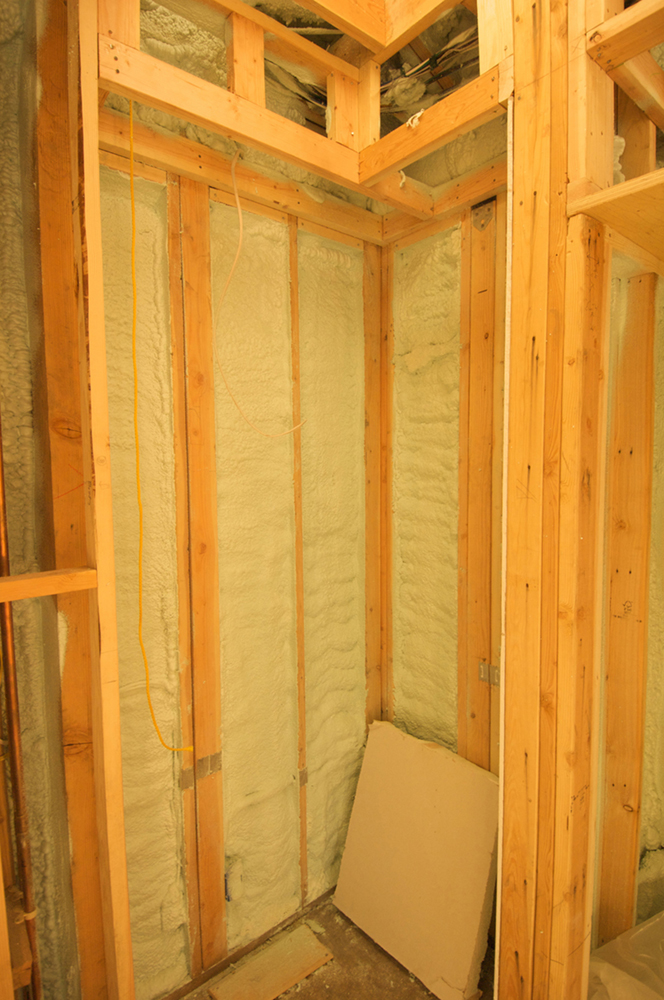Figure 1. Insulation is used to limit the conduction of heat from the inside to the outside (in winters) and from the outside to the inside (in summers). (credit: Giles Douglas)

Your feet feel cold as you walk barefoot across the living room carpet in your cold house and then step onto the kitchen tile floor. This result is intriguing, since the carpet and tile floor are both at the same temperature. The different sensation you feel is explained by the different rates of heat transfer: the heat loss during the same time interval is greater for skin in contact with the tiles than with the carpet, so the temperature drop is greater on the tiles.

Some materials conduct thermal energy faster than others. In general, good conductors of electricity (metals like copper, aluminum, gold, and silver) are also good heat conductors, whereas insulators of electricity (wood, plastic, and rubber) are poor heat conductors. Figure 2 shows molecules in two bodies at different temperatures. The (average) kinetic energy of a molecule in the hot body is higher than in the colder body. If two molecules collide, an energy transfer from the molecule with greater kinetic energy to the molecule with less kinetic energy occurs. The cumulative effect from all collisions results in a net flux of heat from the hot body to the colder body. The heat flux thus depends on the temperature difference ΔT = Thot – Tcold. Therefore, you will get a more severe burn from boiling water than from hot tap water. Conversely, if the temperatures are the same, the net heat transfer rate falls to zero, and equilibrium is achieved. Owing to the fact that the number of collisions increases with increasing area, heat conduction depends on the cross-sectional area. If you touch a cold wall with your palm, your hand cools faster than if you just touch it with your fingertip.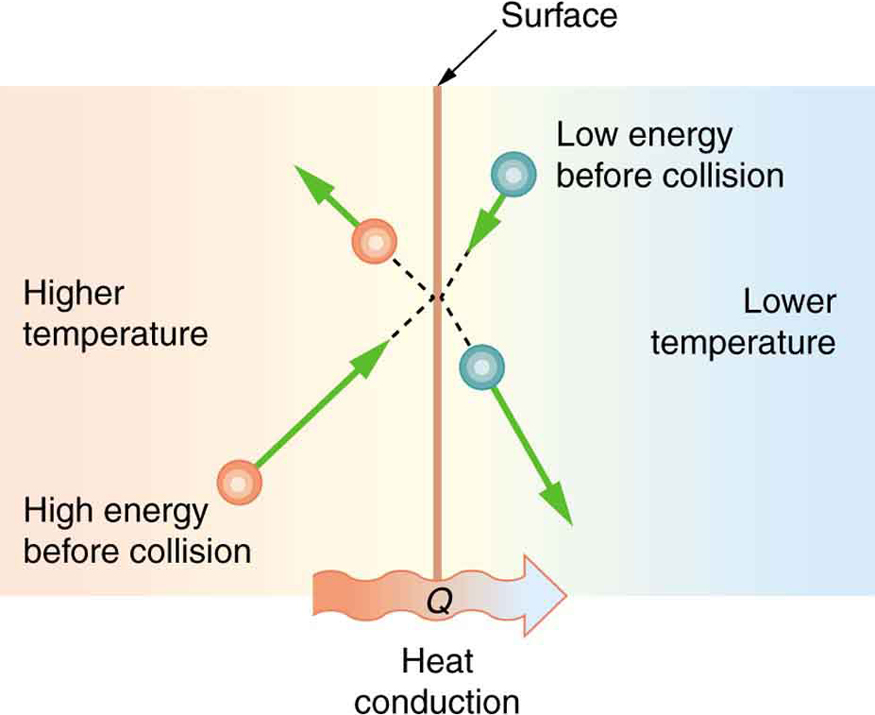Figure 2. The molecules in two bodies at different temperatures have different average kinetic energies. Collisions occurring at the contact surface tend to transfer energy from high-temperature regions to low-temperature regions. In this illustration, a molecule in the lower temperature region (right side) has low energy before collision, but its energy increases after colliding with the contact surface. In contrast, a molecule in the higher temperature region (left side) has high energy before collision, but its energy decreases after colliding with the contact surface.

A third factor in the mechanism of conduction is the thickness of the material through which heat transfers. The figure below shows a slab of material with different temperatures on either side. Suppose that T2 is greater than Tso that heat is transferred from left to right. Heat transfer from the left side to the right side is accomplished by a series of molecular collisions. The thicker the material, the more time it takes to transfer the same amount of heat. This model explains why thick clothing is warmer than thin clothing in winters, and why Arctic mammals protect themselves with thick blubber.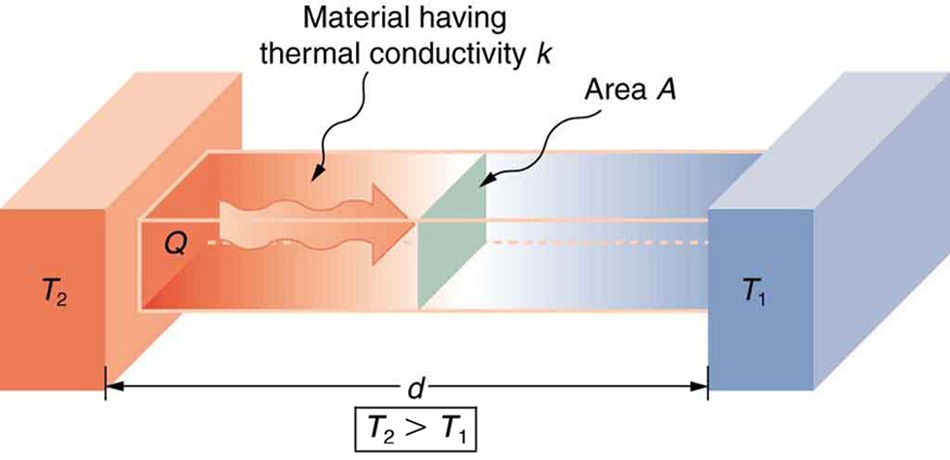Figure 3. Heat conduction occurs through any material, represented here by a rectangular bar, whether window glass or walrus blubber. The temperature of the material is T2 on the left and T1 on the right, where T2 is greater than T1. The rate of heat transfer by conduction is directly proportional to the surface area A, the temperature difference T2−T1, and the substance’s conductivity k. The rate of heat transfer is inversely proportional to the thickness d.

Lastly, the heat transfer rate depends on the material properties described by the coefficient of thermal conductivity. All four factors are included in a simple equation that was deduced from and is confirmed by experiments. The rate of conductive heat transfer through a slab of material, such as the one in Figure 3, is given by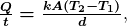where Q/t is the rate of heat transfer in watts or kilocalories per second, is the thermal conductivity of the material, and are its surface area and thickness, as shown in Figure 3, and  T2-T1 is the temperature difference across the slab. Table 3 gives representative values of thermal conductivity.

### Example 1: Calculating Heat Transfer Through Conduction: Conduction Rate Through an Ice Box

A Styrofoam ice box has a total area of 0.950 m and walls with an average thickness of 2.50 cm. The box contains ice, water, and canned beverages at oC. The inside of the box is kept cold by melting ice. How much ice melts in one day if the ice box is kept in the trunk of a car at 35.0 oC?

Strategy

This question involves both heat for a phase change (melting of ice) and the transfer of heat by conduction. To find the amount of ice melted, we must find the net heat transferred. This value can be obtained by calculating the rate of heat transfer by conduction and multiplying by time.

Solution

1. Identify the knowns.
A=0.950 m2 , d=2.50 cm =0.0250 m, T1 = 0 oC, T2= 35.0 oC , t = 1 day =24 hours =86,400 s.
2. Identify the unknowns. We need to solve for the mass of the ice, m. We will also need to solve for the net heat transferred to melt the ice, Q.
3. Determine which equations to use. The rate of heat transfer by conduction is given by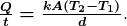4. The heat is used to melt the ice: Q = mL.
5. Insert the known values: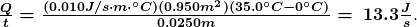6. Multiply the rate of heat transfer by the time  t=1 day= 86,400 s
Q = (Q/t) t = (13.3 J/s )(86,400 s)=1.15 x 106 J
7. Set this equal to the heat transferred to melt the ice: Q = mL  Solve for the mass m.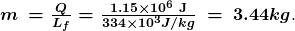Discussion

The result of 3.44 kg, or about 7.6 lbs, seems about right, based on experience. You might expect to use about a 4 kg (7–10 lb) bag of ice per day. A little extra ice is required if you add any warm food or beverages.

Inspecting the conductivities in Table 3 shows that Styrofoam is a very poor conductor and thus a good insulator. Other good insulators include fiberglass, wool, and goose-down feathers. Like Styrofoam, these all incorporate many small pockets of air, taking advantage of air’s poor thermal conductivity.

Substance Thermal conductivity
k  in J / ( s m ºC)
Silver 420
Copper 390
Gold 318
Aluminum 220
Steel iron 80
Steel (stainless) 14
Ice 2.2
Glass (average) 0.84
Concrete brick 0.84
Water 0.6
Fatty tissue (without blood) 0.2
Asbestos 0.16
Plasterboard 0.16
Wood 0.08–0.16
Snow (dry) 0.10
Cork 0.042
Glass wool 0.042
Wool 0.04
Down feathers 0.025
Air 0.023
Styrofoam 0.010
Table 3. Thermal Conductivities of Common Substances1

A combination of material and thickness is often manipulated to develop good insulators—the smaller the conductivity and the larger the thickness , the better. The ratio of d/k will thus be large for a good insulator. The ratio d/k is called the factor. The rate of conductive heat transfer is inversely proportional to R. The larger the value of R, the better the insulation. factors are most commonly quoted for household insulation, refrigerators, and the like—unfortunately, it is still in non-metric units of ft2·°F·h/Btu, although the unit usually goes unstated (1 British thermal unit [Btu] is the amount of energy needed to change the temperature of 1.0 lb of water by 1.0 °F). A couple of representative values are an factor of 11 for 3.5-in-thick fiberglass batts (pieces) of insulation and an factor of 19 for 6.5-in-thick fiberglass batts. Walls are usually insulated with 3.5-in batts, while ceilings are usually insulated with 6.5-in batts. In cold climates, thicker batts may be used in ceilings and walls.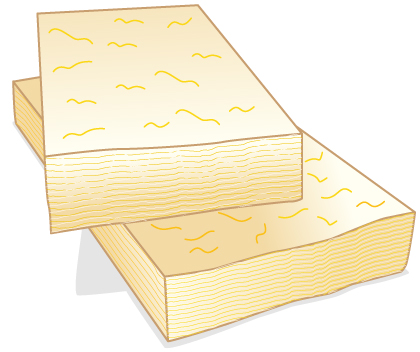Figure 4. The fiberglass batt is used for insulation of walls and ceilings to prevent heat transfer between the inside of the building and the outside environment.

Note that in Table 3, the best thermal conductors—silver, copper, gold, and aluminum—are also the best electrical conductors, again related to the density of free electrons in them. Cooking utensils are typically made from good conductors.

### Example 2: Calculating the Temperature Difference Maintained by a Heat Transfer: Conduction Through an Aluminum Pan

Water is boiling in an aluminum pan placed on an electrical element on a stovetop. The sauce pan has a bottom that is 0.800 cm thick and 14.0 cm in diameter. The boiling water is evaporating at the rate of 1.00 g/s. What is the temperature difference across (through) the bottom of the pan?

Strategy

Conduction through the aluminum is the primary method of heat transfer here, and so we use the equation for the rate of heat transfer and solve for the temperature difference.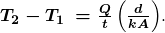Solution

1. Identify the knowns and convert them to the SI units.

The thickness of the pan, d=0.800 cm =8.0 x10 -3 m ,  the area of the pan,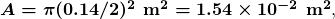and the thermal conductivity,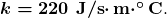2. Calculate the necessary heat of vaporization of 1 g of water: Q = m Lv = (1.00 x 10-3 kg) (2256 x 103 J/kg)  = 2256 J
3. Calculate the rate of heat transfer given that 1 g of water melts in one second:
Q/t = 2256  J/s or  2256 W or 2.26 kW
4. Insert the knowns into the equation and solve for the temperature difference: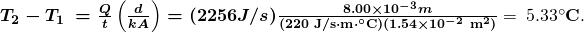Discussion

The value for the heat transfer Q/t = 2256  J/s or  2256 W or 2.26 kW is typical for an electric stove. This value gives a remarkably small temperature difference between the stove and the pan. Consider that the stove burner is red hot while the inside of the pan is nearly 100 0C  because of its contact with boiling water. This contact effectively cools the bottom of the pan in spite of its proximity to the very hot stove burner. Aluminum is such a good conductor that it only takes this small temperature difference to produce a heat transfer of 2.26 kW into the pan.

Conduction is caused by the random motion of atoms and molecules. As such, it is an ineffective mechanism for heat transport over macroscopic distances and short time distances. Take, for example, the temperature on the Earth, which would be unbearably cold during the night and extremely hot during the day if heat transport in the atmosphere was to be only through conduction. In another example, car engines would overheat unless there was a more efficient way to remove excess heat from the pistons.

1: How does the rate of heat transfer by conduction change when all spatial dimensions are doubled?

# Summary

• Heat conduction is the transfer of heat between two objects in direct contact with each other.
• The rate of heat transfer  Q/t (energy per unit time) is proportional to the temperature difference  T2 – T1  and the contact area and inversely proportional to the distance between the objects: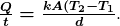### Conceptual Questions

1: Some electric stoves have a flat ceramic surface with heating elements hidden beneath. A pot placed over a heating element will be heated, while it is safe to touch the surface only a few centimeters away. Why is ceramic, with a conductivity less than that of a metal but greater than that of a good insulator, an ideal choice for the stove top?

2: Loose-fitting white clothing covering most of the body is ideal for desert dwellers, both in the hot Sun and during cold evenings. Explain how such clothing is advantageous during both day and night.

### Problems & Exercises

1: (a) Calculate the rate of heat conduction through house walls that are 13.0 cm thick and that have an average thermal conductivity twice that of glass wool. Assume there are no windows or doors. The surface area of the walls is 120 m2 and their inside surface is at 18.0 oC  while their outside surface is at 5.00 oC. (b) How many 1-kW room heaters would be needed to balance the heat transfer due to conduction?

2: The rate of heat conduction out of a window on a winter day is rapid enough to chill the air next to it. To see just how rapidly the windows transfer heat by conduction, calculate the rate of conduction in watts through a  3.00 m2  window that is  0.635 cm thick (1/4 in) if the temperatures of the inner and outer surfaces are 5.00 oC and  -10.0 oC respectively. This rapid rate will not be maintained—the inner surface will cool, and even result in frost formation.

3: Calculate the rate of heat conduction out of the human body, assuming that the core internal temperature is 37.0o, the skin temperature 34.0 oC, the thickness of the tissues between averages 1.00 cm , and the surface area is  1.40 m2.

4: Suppose you stand with one foot on ceramic flooring and one foot on a wool carpet, making contact over an area of 80.0 cm2 with each foot. Both the ceramic and the carpet are 2.00 cm thick and are 10.0 oC on their bottom sides. At what rate must heat transfer occur from each foot to keep the top of the ceramic and carpet at 33.0 oC?

5: A man consumes 3000 kcal of food in one day, converting most of it to maintain body temperature. If he loses half this energy by evaporating water (through breathing and sweating), how many kilograms of water evaporate?

6: (a) A firewalker runs across a bed of hot coals without sustaining burns. Calculate the heat transferred by conduction into the sole of one foot of a firewalker given that the bottom of the foot is a 3.00-mm-thick callus with a conductivity at the low end of the range for wood and its density is  300  kg m3.  The area of contact is 25.0 cm2, the temperature of the coals is 700 oC and the time in contact is 1.00 s.

(b) What temperature increase is produced in the 25.0 cm3 of tissue affected?

(c) What effect do you think this will have on the tissue, keeping in mind that a callus is made of dead cells?

7: (a) What is the rate of heat conduction through the 3.00-cm-thick fur of a large animal having  a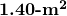surface area? Assume that the animal’s skin temperature is  32.0 o that the air temperature is  -5.00 o, and that fur has the same thermal conductivity as air. (b) What food intake will the animal need in one day to replace this heat transfer?

8: A walrus transfers energy by conduction through its blubber at the rate of 150 W when immersed in  -1.00 owater. The walrus’s internal core temperature is  37.0 oand it has a surface area of  2.00 m2. What is the average thickness of its blubber, which has the conductivity of fatty tissues without blood?

9: Compare the rate of heat conduction through a 13.0-cm-thick wall that has an area of  10.0 m2 and a thermal conductivity twice that of glass wool with the rate of heat conduction through a window that is 0.750 cm thick and that has an area of 2.00 m2 assuming the same temperature difference across each.

10: Suppose a person is covered head to foot by wool clothing with average thickness of 2.00 cm and is transferring energy by conduction through the clothing at the rate of 50.0 W. What is the temperature difference across the clothing, given the surface area is 1.40 m2

11: Some stove tops are smooth ceramic for easy cleaning. If the ceramic is 0.600 cm thick and heat conduction occurs through the same area and at the same rate as computed in Example 2, what is the temperature difference across it? Ceramic has the same thermal conductivity as glass and brick.

12: One easy way to reduce heating (and cooling) costs is to add extra insulation in the attic of a house. Suppose the house already had 15 cm of fiberglass insulation in the attic and in all the exterior surfaces. If you added an extra 8.0 cm of fiberglass to the attic, then by what percentage would the heating cost of the house drop? Take the single story house to be of dimensions 10 m by 15 m by 3.0 m. Ignore air infiltration and heat loss through windows and doors.

13: (a) Calculate the rate of heat conduction through a double-paned window that has a 1.50 m2 area and is made of two panes of 0.800-cm-thick glass separated by a 1.00-cm air gap. The inside surface temperature is  15.0 owhile that on the outside is – 10.0 oC .  (Hint: There are identical temperature drops across the two glass panes. First find these and then the temperature drop across the air gap. This problem ignores the increased heat transfer in the air gap due to convection.)

(b) Calculate the rate of heat conduction through a 1.60-cm-thick window of the same area and with the same temperatures. Compare your answer with that for part (a).

14: Many decisions are made on the basis of the payback period: the time it will take through savings to equal the capital cost of an investment. Acceptable payback times depend upon the business or philosophy one has. (For some industries, a payback period is as small as two years.) Suppose you wish to install the extra insulation in Exercise 12. If energy cost $1.00 per million joules and the insulation was$4.00 per square meter, then calculate the simple payback time. Take the average ΔT for the 120 day heating season to be 15.0 oC.

15: For the human body, what is the rate of heat transfer by conduction through the body’s tissue with the following conditions: the tissue thickness is 3.00 cm, the change in temperature is 2.00 oC. and the skin area is 1.50 m2.  How does this compare with the average heat transfer rate to the body resulting from an energy intake of about 2400 kcal per day? (No exercise is included.)

## Footnotes

1. 1 At temperatures near 0ºC.

## Glossary

R factor
the ratio of thickness to the conductivity of a material
rate of conductive heat transfer
rate of heat transfer from one material to another
thermal conductivity
the property of a material’s ability to conduct heat

### Solutions

1: Because area is the product of two spatial dimensions, it increases by a factor of four when each dimension is doubled  Afinal = (2d)2 =4d2 = 4Ainitial. The distance, however, simply doubles. Because the temperature difference and the coefficient of thermal conductivity are independent of the spatial dimensions, the rate of heat transfer by conduction increases by a factor of four divided by two, or two: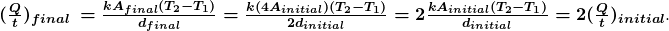Problems & Exercises

1: (a) 1.01 x 10 3b) One

3:  84.0 W

5:  2.59 kg

7: (a) 39.7 W   (b) 820 kcal

9: 35 to 1, window to wall

11. 1.05 x 10 3 K

13: (a) 83 W  (b) 24 times that of a double pane window.

15: 20.0 W, 17.2% of 2400 kcal per day